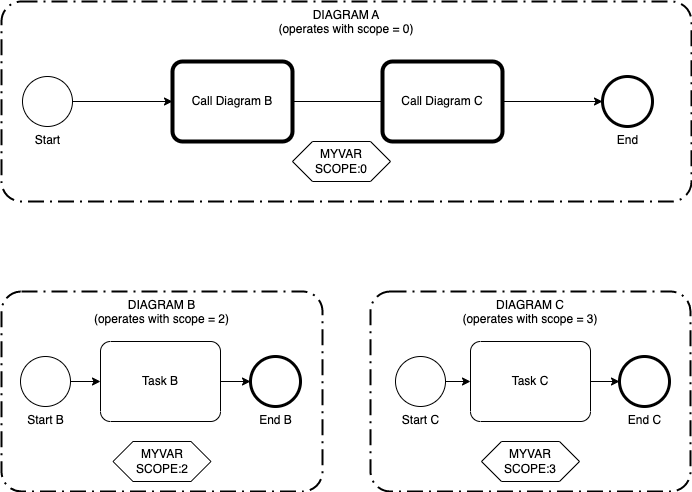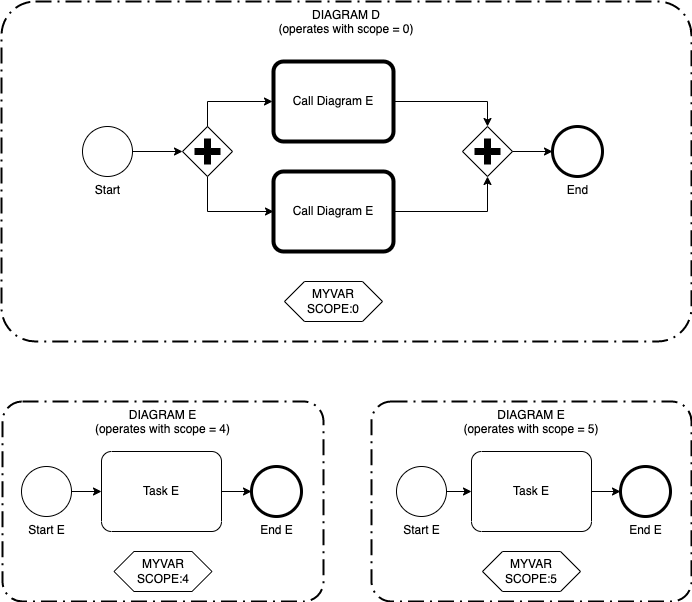You are not looking at the latest version of the documentation. Check it out there.

# Variable Scoping

### Introducing Variable Scoping in v22.2 🆕

Before v22.2, a process instance was executed from a single process diagram, and the developer was responsible for managing the process variables on the diagram. All process variables were accessible by the instance.

Flows for APEX v22.2 introduces Call Activities – where a single process instance can start a process using one process diagram (model) and then can call other diagrams. A process instance could be configured to call the same diagram multiple times – which would result in multiple process variables having the same name present in a single process instance. To keep process variable separate in each invocation of a diagram (within the same process instance), v22.2 introduces variable scoping.

In v22.2, process variables are now scoped at diagram invokation level. Each separate Call Activity creates a new scope for the called diagram to operate in.In the example above, the top level process diagram, diagram A, calls diagram B and diagram C. The initial diagram operates with its scope, scope 0. When Diagram B is called, a new scope is dynamically created at runtime for variables in this diagram – in this case, scope 2. Then control passes back to diagram A, operating at scope 0, before it calls diagram C. when C is called, a new scope is created for variables inside this diagram – in our example, scope 3.

All three diagrams in the example have a process variable `myVar`. As these are on three different diagram calls, scoping results in `MyVar` in Diagram A being a different variable to `MyVar` in Diagram B (scope 2), and different again from `MyVar` in Diagram C (scope 3).To further develop the example, the second diagram shows a top level diagram D which calls Diagram E twice, in parallel.

The process instance starting diagram operates with scope 0; in fact, the top level scope in any process instance is always at scope 0. The instance calls Diagram E twice. One copy of Diagram E operates with scope 4, and the second operates with scope 5. Again, the variable MyVar in each diagram is a different variable.

### Scoping and Single Model Instances.

If your process is defined in a single diagram (model), and does not call another diagram, everything operates at scope 0. The system will operate as it did with previous versions of Flows for APEX, and you don’t need to worry about scoping.

All process variable API calls have scoping as an optional parameter, and if no scope is provided, they default to scope 0.

### Using Scope in a Multi-Model Application

All of the API calls to the process variable system have a signature that allows you to identify the scope of a variable. But how do you keep track of scope? Typically an application will not need to explicitly keep track of the scope that it is operating at. Instead, the application can supply the Subflow ID of the subflow it is currently operating on – and the Flows for APEX engine will use the appropriate scope for that subflow. The engine knows which diagram (or which call of a diagram) every subflow is processing, and can get the appropriate scope information.

If no scope or sublow information information is provided, the scope defaults to scope 0.